# Test: Heat Exchanger Level - 1

## 10 Questions MCQ Test Heat Transfer | Test: Heat Exchanger Level - 1

Description
Attempt Test: Heat Exchanger Level - 1 | 10 questions in 25 minutes | Mock test for Chemical Engineering preparation | Free important questions MCQ to study Heat Transfer for Chemical Engineering Exam | Download free PDF with solutions
QUESTION: 1

### For a heat exchanger the inlet and outlet temperatures of hot and cold fluid areThe type of heat exchanger isTh1 = 150oC Tc1 = 20oCTh2 = 70oC Tc2 = 80oC

Solution: From the given condition of temperature, we see that exit temperature of cold fluid is greater than exit temperature of hot fluid. This is possible only in case of counter flow heat exchanger.

QUESTION: 2

### It is given that for a heat exchangerTc1 and Tc2 are the temperatures of cold fluid at entry and exit respectively and are the temperatures of hot fluid at entry and exit point. If cold fluid has lower heat capacity rate as compared to hot fluid then effectiveness of the heat exchanger is given by

Solution:

Effectiveness, ε = Qact/Qmax

Qact = mhcph(Th1 - Th2) = mccpc (Tc2 - Tc1)

Qmax = (mcp)small(Th1 - Tc1)

Here (mcp)c < (mcp )h

∴ ε (mcp)c (Tc2 - Tc1)/(mcp)c(Th1 - Th2)

⇒ ε = (Tc2 - Tc1)/(Th1 - Tc1)

QUESTION: 3

### Which one of the following heat exchanger gives parallel straight line pattern of temperature distribution for both cold and hot fluid

Solution: For a parallel flow heat exchanger, the temperature profile is always converging except when both fluids are changing phase. But in case of counter flow heat exchanger i

mHcH = mccc

Then

THi - THo = Tco - Tci

∴ THi - Tco = THo - Tci

i.e. θ(x = 0) = θ(x = l)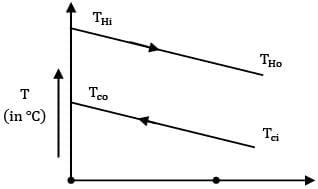x = 0 x = l x

QUESTION: 4

In case of boiler in a steam power plant which of the following configurations gives maximum logarithmic mean temperature difference for given values of heat capacity ratios, inlet and outlet temperatures, over all heat transfer coefficient and heat exchange area

Solution: In a boiler, water changes phase to steam by absorbing heat from hot fluid gasses formed by combustion of coal. During this process the temperature of water remains constant. As a result LMTD of heat exchanger is same in both configurations whether parallel or counter flow.

QUESTION: 5

Consider the following statements The flow configuration in a heat exchanger whether counter flow or otherwise, will not matter if 1. a liquid is evaporating 2. a vapour is condensing 3. mass flow rate of one of the fluids is far greater Which of these statements correct?

Solution: The configuration of the heat exchanger does not matter if one of the fluids is changing phase or capacity rate of one fluid is far greater than capacity rate of the other fluid.

QUESTION: 6

Hot gases enter a heat exchanger at and leave at . The cold air enters at and leaves at . The capacity ratio of the heat exchanger will be

Solution: from energy balance we have,

Ch(Thi - The) = Cc(Tce - Tci)

⇒ Ch/Cc = (140-40)/(200-150) = 100/50 = 2

Or

Cc/Ch = 0.5

QUESTION: 7

What does NTU indicate?

Solution:

NTU = UA/Cmin

∵ NTU ∝ A, it indicates size of heat exchanger

QUESTION: 8

For a balanced counter flow heat exchanger (i.e. mh ch = mc cc), NTU is equal to 1.0. What is the effectiveness of the heat exchanger?

Solution: For a balanced counter flow heat exchanger effectiveness is given as

ε = NTU/1+NTU = 1/1+1

⇒ ε = 1/2

⇒ ε = 0.5

QUESTION: 9

Which of the following temperature profiles represents a counter flow exchanger with heat capacity rate of hot fluid (CH) greater than heat capacity rate of cold fluid (CC)

(A)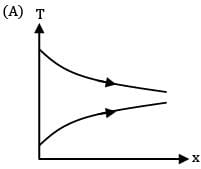(B)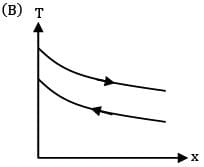(C)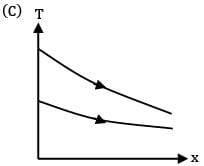(D)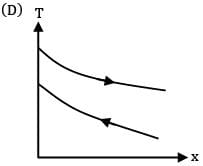Solution: For a counter flow heat exchanges, the two fluids flow in opposite directions. Also by steady state flow energy equation

CH(THi - THo) = Cc(Tco - Tci)

Given CH > CC

∴ THi -THo < />co -TCi

∴ THi -TCo < />Ho -TCi

∴ ፀ( x = 0) < ፀ(="" x="" />

Thus correct profile is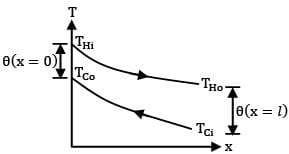QUESTION: 10

Match List-I with List-II and select the correct answer using the code given below the lists

List-I

a. Number of transfer units

b. Periodic flow heat exchangers

c. Phase change

d. Deposition on heat exchanger surface

List-II

1. Regenerators

2. Fouling factor

3. A measure of heat exchanger size

4. Condensers

Codes: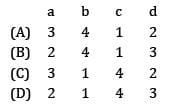Solution:Use Code STAYHOME200 and get INR 200 additional OFF Use Coupon Code